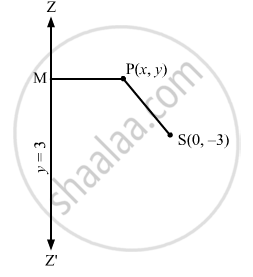Advertisement Remove all ads

# Find the Equation of the Parabola If the Focus is at (0, −3) and the Vertex is at (0, 0) - Mathematics

Find the equation of the parabola if

the focus is at (0, −3) and the vertex is at (0, 0)

Advertisement Remove all ads

#### Solution

In a parabola, the vertex is the mid-point of the focus and the point of intersection of the axis and the directrix.

Let (x1, y1) be the coordinates of the point of intersection of thIt is given that the vertex and the focus of a parabola are (0, 0) and (0, −3), respectively.

Thus, the slope of the axis of the parabola cannot be defined.

Slope of the directrix = 0

Let the directrix intersect the axis at (rs). e axis and directrix.

∴ $\frac{r + 0}{2} = 0, \frac{s - 3}{2} = 0$
$\Rightarrow r = 0, s = 3$

∴ Required equation of directrix:

$y = 3$

Let (xy) be any point on the parabola whose focus is S (0, −3) and the directrix is $y = 3$Draw PM perpendicular to $y = 3$

Then, we have:

$SP = PM$
$\Rightarrow S P^2 = P M^2$
$\Rightarrow \left( x - 0 \right)^2 + \left( y + 3 \right)^2 = \left( \frac{y - 3}{\sqrt{1}} \right)^2$
$\Rightarrow x^2 + y^2 + 6y + 9 = y^2 - 6y + 9$
$\Rightarrow x^2 = - 12y$

Is there an error in this question or solution?
Advertisement Remove all ads

#### APPEARS IN

RD Sharma Class 11 Mathematics Textbook
Chapter 25 Parabola
Exercise 25.1 | Q 3.2 | Page 24
Advertisement Remove all ads

#### Video TutorialsVIEW ALL 

Advertisement Remove all ads
Share
Notifications

View all notifications

Forgot password?